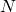# ILP Part 46 — Gray Code

This is the forty sixth part of the ILP series. For your convenience you can find other parts in the table of contents in Part 1 – Boolean algebra

Hi. Today we are going to generate Gray code using ILP. I am not going to cover theory here, you can find it on Wikipedia.

We want to generatecodes whereis power of two. First, we definenon-negative integer variables. Next, we add constraints that make all variables different. Finally, we need to make sure that variables represent Gray codes.

For each pair of variables we first calculate their unsigned magnitude representation, next we xor digits at the same positions from both of the representations. Next, we sum xors and add constraint that this sum must be equal to one.

The code solves the problem: